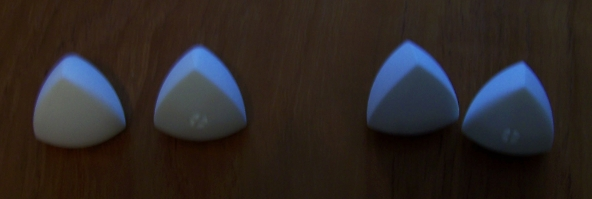## The Reuleaux Tetrahedron

Why are manhole covers round? The manhole covers are round because the manholes are round. Duh! But the cute mathematical answer is that the round shapes are better than many other shapes because a round cover can’t fall into a round hole. If we assume that the hole is the same shape as the cover but slightly smaller, then it is true that circular covers can’t fall into their holes. But there are many other shapes with this property. They are called the shapes of constant width.Given the width, the shape with the largest area is not surprisingly a circle. The shape with the smallest area and a given constant width is a Reuleaux Triangle. Here is how to draw a Reuleaux triangle. Draw three points that are equidistant from each other at distance d. Then draw three circles of radius d with the centers at given points. The Reuleaux triangle is the intersection of these three circles.

Can we generalize this to 3d? What would be an analogue of a Reuleaux Triangle in 3d? Of course, it is a Reuleaux Tetrahedron: Take four points at the vertices of a regular tetrahedron; take a sphere at each vertex with the radius equal to the edge of the tetrahedron; intersect the four spheres.

Is this a shape of the constant width? Many people mistakenly think that this is the case. Indeed, if you squeeze the Reuleaux tetrahedron between two planes, one of which touches a vertex and another touches the opposite face of the curvy tetrahedron, then the distance between them is equal to d: the radius of the circle. This might give you the impression that this distance is always d. Not so. If you squeeze the Reuleaux tetrahedron between two planes that touch the opposite curvy edges, the distance between these planes will be slightly more than d. To create a shape of constant width you need to shave off the edges a bit.Theoretically you can shave the same amount off every edge to get to a surface of constant width. But this is not the cool way to do it. The cool way is to shave a bit more but only from one edge of the pair of opposite edges. You can get two different figures this way: one that has three shaved edges forming a triangle, and the other, where three shaved edges share a vertex. These two bodies are called Meissner bodies and they are conjectured to be shapes of the constant width with the smallest volume.

On the picture I have two copies of a pair of Meissner bodies. The two left ones have three edges that share a vertex shaved off. The very left shape gives a top view of this vertex and the solid next to it has its bottom with holes looking forward. The two shapes on the right show the second Meissner body in two different positions.

I recently discovered a TED-Ed video about manhole covers. It falsely claims that the Reuleaux tetrahedron has constant width. I wrote to TED-Ed, to the author, and posted a comment on the discussion page. There was no reaction. They either should remove the video or have an errata page for it. Knowingly keeping a video with an error that is being viewed by thousands of people is irresponsible.

Share: